SSAT Middle Level Math : Quadrilaterals

Example Questions

← Previous 1 3 4 5 6 7 8 9 13 14

Florence wants to build a fence to surround her square garden.  The garden has an area of 16 square yards. How much fencing will she need?Explanation:

So this question requires you to know the formula for area for a square which is side squared.

Thus,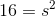, so one side is the square root of 16, or 4.

To figure out the perimeter, add up all of the sides of the square which are all the same (4).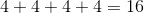A square has perimeter 89.4 centimeters. What is the length of one side?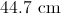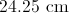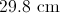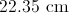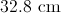Explanation:

A square has four sides of equal measure, so divide its perimeter by 4: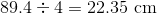A square has perimeter 16 feet 4 inches. What is the length of one side?

4 feet 5 inches

3 feet 11 inches

3 feet 9 inches

4 feet 3 inches

4 feet 1 inch

4 feet 1 inch

Explanation:

One foot is equal to 12 inches, so the perimeter 16 feet 4 inches is equal to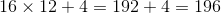inches.

An square has four sides of equal measure, so divide its perimeter by 4: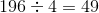To convert 49 inches to feet and inches: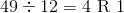One side measures 4 feet 1 inch.

The length of one side of a square is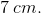What is the perimeter of this square?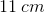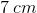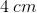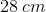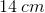Explanation:

The perimeter of a shape is the distance around the outside boundry of that shape.

In order to find the perimeter, add up the lengths of the sides of that shape.

Because a square has four equal sides, add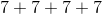to find the perimeter. Equivalently, multiply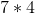.

Find the perimeter of the square in the figure.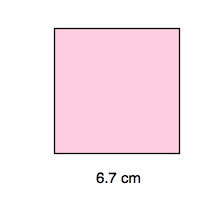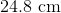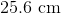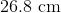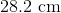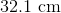Explanation:

Finding perimeter is easiest with squares. Perimeter is the distance around the outside of the figure. There are two good ways to find the perimeter. The first and longer method of finding perimeter is simply to add up the length of each side. Thus, we would take the length of top of the square and add it to the length of the right side. We'd continue all the way around. The formula for this method could be written like so:

top + right side + bottom + left side = perimeter.

Since a square has equal sides all around, we could also write the formulalike so: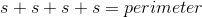The second and easier way of finding perimeter for a square is a simplification of the first method. Instead of adding the same number four times we could simply multiply by 4. Written as a formula, this would look like this: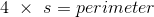For the this question, your work should then look like one of these two options: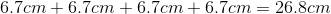or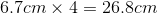Find the perimeter of the square in the figure.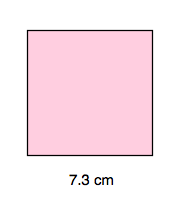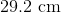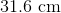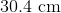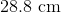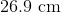Explanation:

Finding perimeter is easiest with squares. Perimeter is the distance around the outside of the figure. There are two good ways to find the perimeter. The first and longer method of finding perimeter is simply to add up the length of each side. Thus, we would take the length of top of the square and add it to the length of the right side. We'd continue all the way around. The formula for this method could be written like so:

top + right side + bottom + left side = perimeter.

Since a square has equal sides all around, we could also write the formulalike so:The second and easier way of finding perimeter for a square is a simplification of the first method. Instead of adding the same number four times we could simply multiply by 4. Written as a formula, this would look like this:For the this question, your work should then look like one of these two options: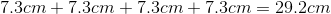or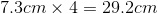Example Question #1 : How To Find The Perimeter Of A Square

Find the perimeter of the square in the figure.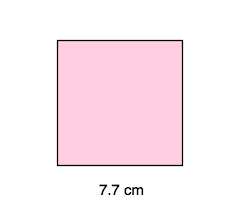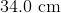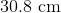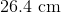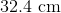Explanation:

Finding perimeter is easiest with squares. Perimeter is the distance around the outside of the figure. There are two good ways to find the perimeter. The first and longer method of finding perimeter is simply to add up the length of each side. Thus, we would take the length of top of the square and add it to the length of the right side. We'd continue all the way around. The formula for this method could be written like so:

top + right side + bottom + left side = perimeter.

Since a square has equal sides all around, we could also write the formulalike so:The second and easier way of finding perimeter for a square is a simplification of the first method. Instead of adding the same number four times we could simply multiply by 4. Written as a formula, this would look like this:For the this question, your work should then look like one of these two options: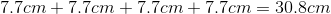or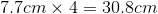Find the perimeter of the square in the figure.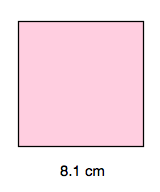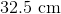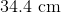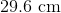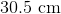Explanation:

Finding perimeter is easiest with squares. Perimeter is the distance around the outside of the figure. There are two good ways to find the perimeter. The first and longer method of finding perimeter is simply to add up the length of each side. Thus, we would take the length of top of the square and add it to the length of the right side. We'd continue all the way around. The formula for this method could be written like so:

top + right side + bottom + left side = perimeter.

Since a square has equal sides all around, we could also write the formulalike so:The second and easier way of finding perimeter for a square is a simplification of the first method. Instead of adding the same number four times we could simply multiply by 4. Written as a formula, this would look like this:For the this question, your work should then look like one of these two options: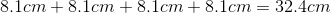or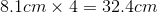Find the perimeter of the square in the figure.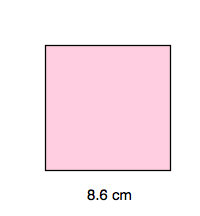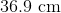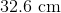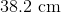Explanation:

Finding perimeter is easiest with squares. Perimeter is the distance around the outside of the figure. There are two good ways to find the perimeter. The first and longer method of finding perimeter is simply to add up the length of each side. Thus, we would take the length of top of the square and add it to the length of the right side. We'd continue all the way around. The formula for this method could be written like so:

top + right side + bottom + left side = perimeter.

Since a square has equal sides all around, we could also write the formulalike so:The second and easier way of finding perimeter for a square is a simplification of the first method. Instead of adding the same number four times we could simply multiply by 4. Written as a formula, this would look like this:For the this question, your work should then look like one of these two options: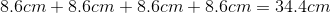or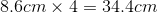Find the perimeter of the square in the figure.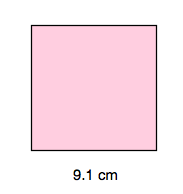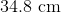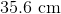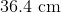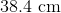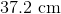Explanation:

Finding perimeter is easiest with squares. Perimeter is the distance around the outside of the figure. There are two good ways to find the perimeter. The first and longer method of finding perimeter is simply to add up the length of each side. Thus, we would take the length of top of the square and add it to the length of the right side. We'd continue all the way around. The formula for this method could be written like so:

top + right side + bottom + left side = perimeter.

Since a square has equal sides all around, we could also write the formulalike so:The second and easier way of finding perimeter for a square is a simplification of the first method. Instead of adding the same number four times we could simply multiply by 4. Written as a formula, this would look like this:For the this question, your work should then look like one of these two options: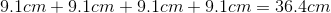or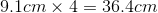← Previous 1 3 4 5 6 7 8 9 13 14